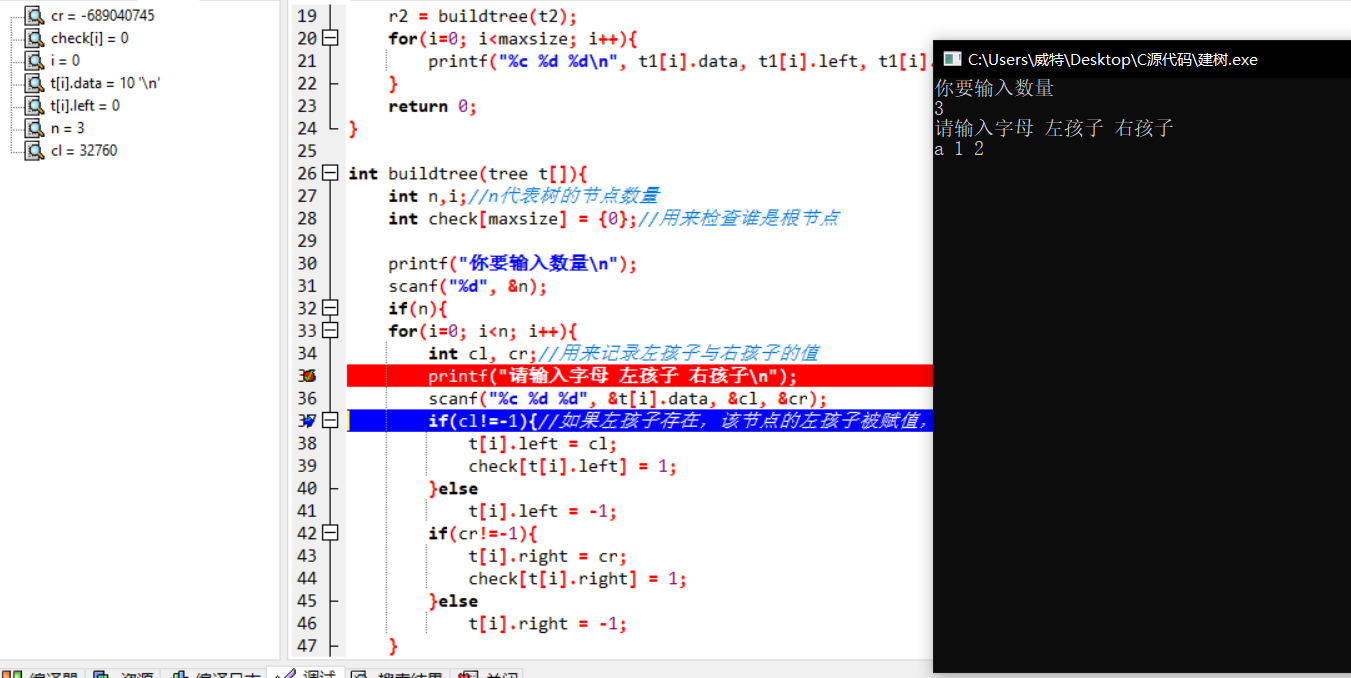c语言静态链表无法成功赋值

** scanf("%c %d %d", &t[i].data, &cl, &cr);**这一行出了问题。无法正常赋值，不知道怎么回事。调试如下：``````#include<stdio.h>

#define maxsize 5
#define Null -1

struct treenode{
char data;
int left;
int right;
};
typedef struct treenode tree;
int buildtree(tree t[]);

int main(){
tree t1[maxsize], t2[maxsize];//用结构体定义了两个结构数组，用来存储树
int r1, r2, i;//r1 r2 代表的是t1，t2结构数组中根节点的下标
r1 = buildtree(t1);
r2 = buildtree(t2);
for(i=0; i<maxsize; i++){
printf("%c %d %d\n", t1[i].data, t1[i].left, t1[i].right);
}
return 0;
}

int buildtree(tree t[]){
int n,i;//n代表树的节点数量
int check[maxsize] = {0};//用来检查谁是根节点

printf("你要输入数量\n");
scanf("%d", &n);
if(n){
for(i=0; i<n; i++){
int cl, cr;//用来记录左孩子与右孩子的值
printf("请输入字母 左孩子 右孩子\n");
**      scanf("%c %d %d", &t[i].data, &cl, &cr);**
printf("%c %d %d", t[i].data, cl, cr);
if(cl!=-1){//如果左孩子存在，该节点的左孩子被赋值，并且记录到check数组，来证明这不是一个孤儿。
t[i].left = cl;
check[t[i].left] = 1;
}else
t[i].left = -1;
if(cr!=-1){
t[i].right = cr;
check[t[i].right] = 1;
}else
t[i].right = -1;
}
}
else
return -1;

for(i=0; i<maxsize; i++)
if(!check[i])
break;
return i;
}
``````

2个回答

``````scanf("%d", &n);
``````

``````scanf("%c %d %d", &t[i].data, &cl, &cr);

``````

``````scanf("%d\n", &n);
.....
scanf("%c %d %d\n", &t[i].data, &cl, &cr);
``````

``````fflush (stdin);
``````Weite_Zhang scanf("%c %c %c", &ele, &l, &r); fflush(stdin); trec[i].element = ele;//接受本节点字母 if(l!='-') { trec[i].left = l - '0';//注意此处不要写成 'l'-0 如此结果便成了 108-0=108 check[trec[i].left] = 1; }在我使用l-’0‘之后，这个程序就不能正常输入了。这是怎么回事呢？
4 个月之前 回复Weite_Zhang 我想继续追问一个问题：
4 个月之前 回复Weite_Zhang 感谢回复，问题已经解决了
5 个月之前 回复5 个月之前 回复5 个月之前 回复

fflush(stdin); //前面加上
scanf("%c%d%d", &(t[i].data), &cl, &cr);